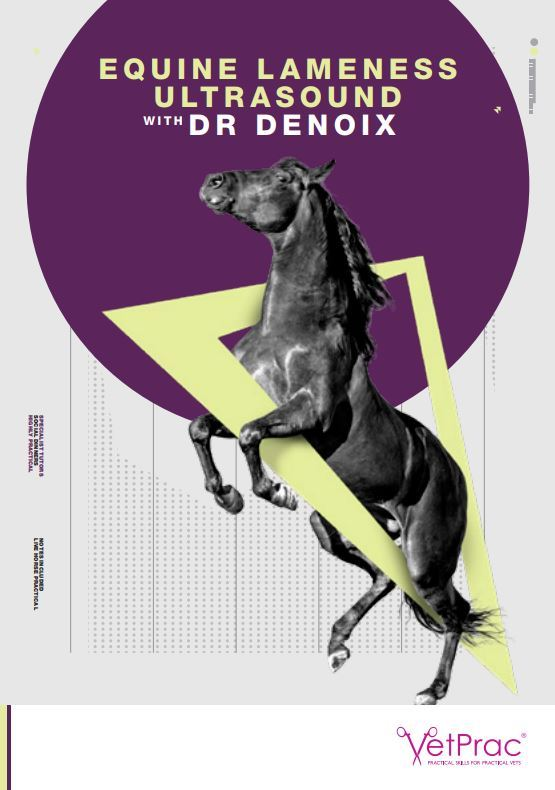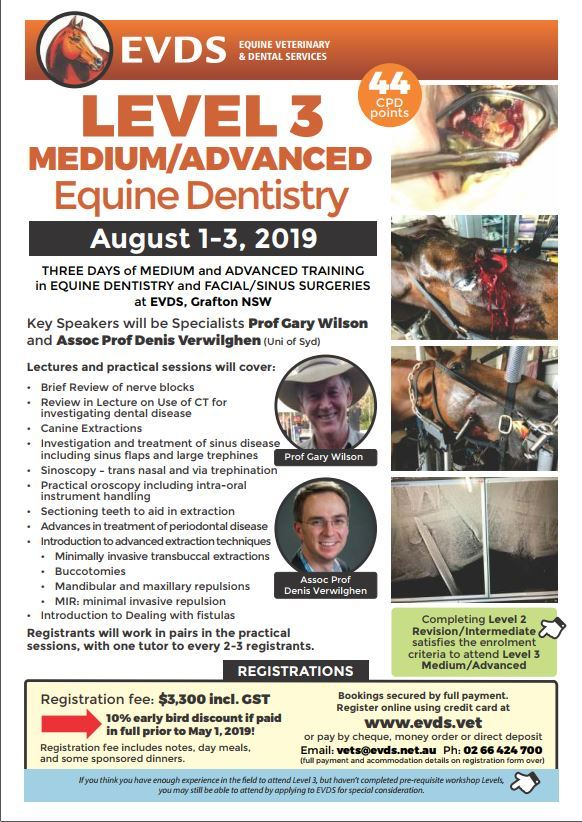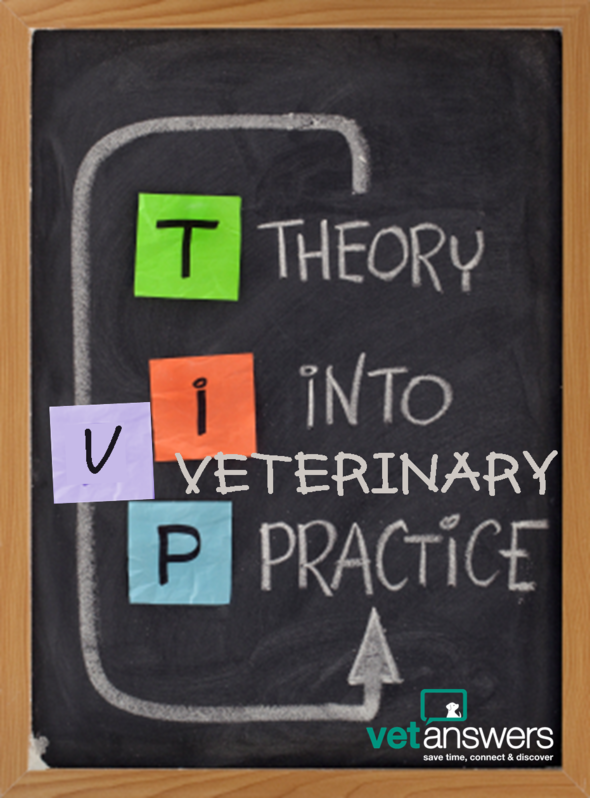Like   Tweet   Pin   in

Hi Member,

This week has been a crazy mad rush because on Monday, we leave for the small island of Nusa Lembongan, just off Bali, for 10 days. I wanted to have as much organised as possible before I left, however, you may notice that the next couple of eNews may not be quite as jammed packed as usual.

But I'm not on the plane yet, so today we have Part 12 in our special blog post series: So many other things - The reality of working in veterinary practice - Support system ascending - which continues the examination of mental health and support networks within the veterinary industry.

I've also updated our What's on in 2019? Veterinary CPD Calendar for June. Check out both the pdf and online version to find all the CPD on offer for you and your team :)

I'd also like to welcome back to our Community Heart Harmony Communications and to welcome for the first time MARS Petcare College - both with a Free Listing in our Business Directory.

Finally - a bit of a heads-up! If you were thinking of adding Laser Therapy to your business, then now is the time to make the phone call as Vetsone is offering a 20% discount to Vetanswers members, but only until the 30th June - find out more below.

## Save Time, Connect & Discover!table div table+table+table+table div table{width:100%;padding:0}table div table+table+table+table div table img{width:96.23%;padding:0;float:none}table div table+table+table+table div table td{width:100%;padding:0 1.88% 18px}/* styles */## So Many Other Things: The Reality of Veterinary Practice Part 12 - Support System Ascending

The next part in our popular blog series about the realities of working in veterinary practice - this one continues on the mental health and support theme...

## 3 Things I bet you didn't know about... Veterinary Apps

Veterinary Applications offer the only Australian iPad Apps specifically designed for use during veterinary consults. If you'd like to improve client communication, compliance & consistency in your practice, then check out what they're experts at & how they can help you...

 table div table+table+table+table+table+table+table+table div table{width:100%;padding:0}table div table+table+table+table+table+table+table+table div table img{width:96.23%;padding:0;float:none}table div table+table+table+table+table+table+table+table div table td{width:100%;padding:0 1.88% 18px}/* styles */# Heart Harmony Communications

Heart Harmony Communications can help to get you online without the overwhelm with fabulous websites and web content that your clients and Google will love.

Read a blog post from HHC which we shared this week: Perfectionism: You Can’t Edit a Blank Page

# MARS Petcare College

MARS Petcare College is a training program for people who work in the pet industry. Veterinarians & Veterinary Nurses can both earn 10 CPD by completing their online course.

 table div table+table+table+table+table+table+table+table+table+table+table+table div table{width:100%;padding:0}table div table+table+table+table+table+table+table+table+table+table+table+table div table img{width:96.23%;padding:0;float:none}table div table+table+table+table+table+table+table+table+table+table+table+table div table td{width:100%;padding:0 1.88% 18px}/* styles */## UPDATED FOR JUNE! What's on in 2019? Veterinary CPD Calendar...

 table div table+table+table+table+table+table+table+table+table+table+table+table+table+table div table{width:100%;padding:0}table div table+table+table+table+table+table+table+table+table+table+table+table+table+table div table table{padding:0;float:left!important;width:50.754%!important}table div table+table+table+table+table+table+table+table+table+table+table+table+table+table div table table+table td,table div table+table+table+table+table+table+table+table+table+table+table+table+table+table div table td{padding-left:0;padding-right:0}table div table+table+table+table+table+table+table+table+table+table+table+table+table+table div table table td{padding-left:0;padding-right:20px}table div table+table+table+table+table+table+table+table+table+table+table+table+table+table div table table+table{float:left!important;width:49.246%!important}/* styles */

## Featuring.....# VetPrac Workshop: Equine Lameness Ultrasound with Dr Denoix

4 to 5 July: Beginner-Intermediate (Distal Limbs) Only 5 places left
6 to 7 July: Intermediate-Advanced (Proximal Limbs & Spine) NOW FULL
Where: UQ VETS Equine Specialist Hospital, Gatton, QLD

# ~~~~~~# EVDS Workshop: Level 3 Medium / Advanced Equine Dentistry

1 to 3 August, South Grafton
Key Speakers: Specialists Prof Gary Wilson and Assoc Prof Denis Verwilghen (Uni of Syd)

Extend and refine your equine dentistry and facial/sinus surgery skills with 3 days of medium and advanced training in equine dentistry and facial/sinus surgeries.

 table div table+table+table+table+table+table+table+table+table+table+table+table+table+table+table+table+table+table+table div table{width:100%;padding:0}table div table+table+table+table+table+table+table+table+table+table+table+table+table+table+table+table+table+table+table div table img{width:96.23%;padding:0;float:none}table div table+table+table+table+table+table+table+table+table+table+table+table+table+table+table+table+table+table+table div table td{width:100%;padding:0 1.88% 18px}/* styles */# Deductions you can claim...

Work-related expenses
1. You must have spent the money yourself and weren't reimbursed
2. It must directly relate to earning your income
3. You must have a record to prove it.

 table div table+table+table+table+table+table+table+table+table+table+table+table+table+table+table+table+table+table+table+table+table+table+table div table{width:100%;padding:0}table div table+table+table+table+table+table+table+table+table+table+table+table+table+table+table+table+table+table+table+table+table+table+table div table img{width:96.23%;padding:0;float:none}table div table+table+table+table+table+table+table+table+table+table+table+table+table+table+table+table+table+table+table+table+table+table+table div table td{width:100%;padding:0 1.88% 18px}/* styles */## Featured Product: Special Offer for Vetanswers Members ends 30 June

 table.module-24{width:94.72%;padding:0}table div table+table+table+table+table+table+table+table+table+table+table+table+table+table+table+table+table+table+table+table+table+table+table+table+table div table{width:94.72%;float:none;margin-left:auto;margin-right:auto;padding:0}table div table+table+table+table+table+table+table+table+table+table+table+table+table+table+table+table+table+table+table+table+table+table+table+table+table div table a{border:0 none;text-decoration:none}table div table+table+table+table+table+table+table+table+table+table+table+table+table+table+table+table+table+table+table+table+table+table+table+table+table div table img{width:100%!important;border:0 none;text-decoration:none}table div table+table+table+table+table+table+table+table+table+table+table+table+table+table+table+table+table+table+table+table+table+table+table+table+table div table td{width:100%;padding:0}/* styles */
 table div table+table+table+table+table+table+table+table+table+table+table+table+table+table+table+table+table+table+table+table+table+table+table+table+table+table+table div table{width:100%;padding:0}table div table+table+table+table+table+table+table+table+table+table+table+table+table+table+table+table+table+table+table+table+table+table+table+table+table+table+table div table img{width:96.23%;padding:0;float:none}table div table+table+table+table+table+table+table+table+table+table+table+table+table+table+table+table+table+table+table+table+table+table+table+table+table+table+table div table td{width:100%;padding:0 1.88% 18px}/* styles */# Veterinary labor's wild swings - The difference between oversupply and excess capacity

## By Michael Dicks & Melissa Maddux

This week the 6th in the series of posts by economist Michael Dicks discussing the economics behind labor fluctuations in the veterinary industry.

This post discusses the labor situation in the US veterinary industry, however it still includes great information and suggestions that can also be applied to the Australian industry.

"Training future veterinarians in numbers that match the forecasted demand for services; being more flexible in defining the number of hours in an individual's workweek; and managing for changes in economic conditions can help minimize the variability in the supply of veterinary labor."

The important distinction between excess capacity and oversupply:
"Excess capacity is the ability to provide more services than are demanded at a specific price. In a situation of excess capacity, demand for veterinary services could be heightened by lowering prices, adding locations and/or offering an expanded range of services. Oversupply, by contrast, suggests that even if services were free, their availability would exceed the need."

 table div table+table+table+table+table+table+table+table+table+table+table+table+table+table+table+table+table+table+table+table+table+table+table+table+table+table+table+table+table+table+table div table{width:100%;padding:0}table div table+table+table+table+table+table+table+table+table+table+table+table+table+table+table+table+table+table+table+table+table+table+table+table+table+table+table+table+table+table+table div table img{width:96.23%;padding:0;float:none}table div table+table+table+table+table+table+table+table+table+table+table+table+table+table+table+table+table+table+table+table+table+table+table+table+table+table+table+table+table+table+table div table td{width:100%;padding:0 1.88% 18px}/* styles */table div table+table+table+table+table+table+table+table+table+table+table+table+table+table+table+table+table+table+table+table+table+table+table+table+table+table+table+table+table+table+table+table+table+table div table{width:100%;padding:0}table div table+table+table+table+table+table+table+table+table+table+table+table+table+table+table+table+table+table+table+table+table+table+table+table+table+table+table+table+table+table+table+table+table+table div table img{width:96.23%;padding:0;float:none}table div table+table+table+table+table+table+table+table+table+table+table+table+table+table+table+table+table+table+table+table+table+table+table+table+table+table+table+table+table+table+table+table+table+table div table td{width:100%;padding:0 1.88% 18px}/* styles */table div table+table+table+table+table+table+table+table+table+table+table+table+table+table+table+table+table+table+table+table+table+table+table+table+table+table+table+table+table+table+table+table+table+table+table+table+table+table div table{width:100%;padding:0}table div table+table+table+table+table+table+table+table+table+table+table+table+table+table+table+table+table+table+table+table+table+table+table+table+table+table+table+table+table+table+table+table+table+table+table+table+table+table div table img{width:96.23%;padding:0;float:none}table div table+table+table+table+table+table+table+table+table+table+table+table+table+table+table+table+table+table+table+table+table+table+table+table+table+table+table+table+table+table+table+table+table+table+table+table+table+table div table td{width:100%;padding:0 1.88% 18px}/* styles */